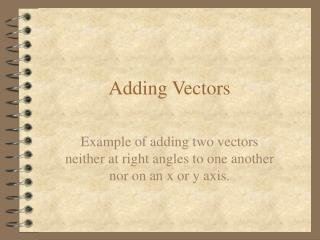DownloadDownload PresentationTélécharger la présentation- - - - - - - - - - - - - - - - - - - - - - - - - - - E N D - - - - - - - - - - - - - - - - - - - - - - - - - - -
##### Presentation Transcript

1. Adding Vectors Example of adding two vectors neither at right angles to one another nor on an x or y axis.

2. The Process: • First draw the vectors on an x:y axis, showing them attached head to tail. • Second, determine the x and y components of V1. • Third, determine the x and y components of V2. • Fourth, Add the x components of the vectors together. • Fifth, Add the y components of the vectors together. • Sixth, Use the sum of the x components as the x component of the resultant vector; Use the sum of the y components as the y component of the resultant vector. • Seventh, proceed to “add” the resultant’s x and y values.

3. The Problem:

4. Resolve the 1st vector into its x and y components. V1y = V1 * Sin 60 or V1 * Cos 30 = 0.866 Km, N V1x = V1 * Cos 60 or V1 * Sin 30 = 0.5 km, E

5. Resolve the 2nd vector into its x and y components. V2y = V2 * Sin 30 or V2 * Cos 60 = 1 Km, N V2x = V2 * Cos 30 or V2 * Sin 60 = 1.732 Km, E

6. Next, add the components. V1y + V2y = 0.866 Km + 1.000 Km = 1.866 Km, N V1x + V2x = 0.500 Km + 1.732 Km = 2.232 Km, E

7. Determine the resultant: 1st use c^2 = a^2 + b^2 c = (a^2 + b^2)^(1/2) c = [(1.866 km)^2 + (2.232 km)^2]^(1/2) So c = 2.909 km 2nd use Angle = Inv Tan (Ry / Rx) = Inv Tan (1.886 km / 2.232 km) = 39.9 degrees; The direction is N of E. So R (the resultant) is equal to 2.909 Km, 39.9 deg N of E or 2.909 Km, 50.1 deg from N, or E of N